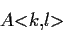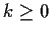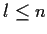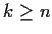Next: 2.1.2 Lists Up: 2.1.1 Arrays Previous: 2.1.1 Arrays

### 2.1.1.1 Recipes for Processing Arrays

Arrays do not have the same internal structure as lists: an array of length n does not contain an array of length n-1 as a component. Hence, the structural design recipes for processing lists do not directly apply to lists. But it is easy to mimic the structural decomposition of lists as follows. Given an array

A = a0, ..., an-1

we define the slicewhereandas the sequence of elements

ak, ..., al-1

Since array slices are not arrays, we cannot pass them to helper functions as array values. Instead we must pass three values in the general case: the array A, and the two int bounds k and l. Fortunately, in most list processing recipes one of two bounds is always fixed (either k at 0, or l at n), so we only need to pass two values.

Assume that we want write a static method int sum takes an argument a of type int[] and returns the sum of the elements of a. If we were summing the elements of a list instead of an array, we could use the natural recursion scheme on lists to reduce summing a compound list (a Cons) to summing its tail (the rest component of the list). (See Section 1.6.2.) We can use exactly the same scheme to sum an array provided that we use array slices instead of array values. To process slices, we must write a help method sumHelp that takes arguments a and k of type int[] and int respectively and returns the sum of the elements in the array slice a<k,n> where n is the length of the array a. An empty slice corresponds to the case where. A compound slice corresponds to the case where k < n.

The following Java code implements the sum method

class ArrayUtil {
public static int sum(int[] a) {
// returns a + ... + a[a.length-1]
return sumHelp(a,0);
}
public static int sumHelp(int[] a, int k) {
// given 0 <= k < a.length
// returns a[k] +  ... +  a[a.length-1]
if (k >= a.length) then return 0;
else return	a[k] + sumHelp(a, k+1);
}
}


From the standpoint of computational efficiency, neither the natural recursion program or the equivalent program on array slices written above is optimal because neither one is tail-recursive. A method definition is tail-recursive if recursive calls only appear in tail-position'', the last operation before returning from the method. In Scheme, the standard recipe for converting such a computation to tail recursive form involves writing a help function with an accumulating parameter and summing the elements in the opposite order (left-to-right instead of right-to-left). We can convert our array slice solution to tail-recursive form using essentially the same transformation.

The following Java code implements a tail-recursive solution using array slices:

class ArrayUtil {
public static int sum(int[] a) {
return tailSumHelp(a,0,0);
}
public static int tailSumHelp(int[] a, int k, int accum) {
// given 0 <= k < a.length
// returns accum + a[k] +  ... +  a[a.length-1]
if (k >= a.length) then return accum;
else return	tailSumHelp(a, k+1, accum+a[k]);
}
}


In languages that do not support the efficient translation of tail-recursive procedures to machine code, tail recursive (also called iterative) computations must be expressed in the more restrictive framework of for and while loops to produce efficient code. A tail-recursive procedure is a more general framework for expressing iterative computations than structured loops! In contrast to structured loops, tail-recursive procedures gracefully accommodate iterations with exit conditions; each procedure return clause that is not a tail-recursive call is an exit. To translate the an iterative program expressed using tail recursion to one expressed using a loop, the corresponding loop construction must have multiple exit jumps (implemented as break or go to).

Java has three familiar looping constructs: while loops, do .. while loops, C-style for loops. The first two constructs are completely standard. A while loop has syntax:

while (test) do statement
where statement is usually a block. A block is simply a sequence of local variable declarations and statements enclosed in braces. The test expression must have boolean type. A do while loop has syntax:
do statement while (test);
The only different between the two looping constructs is the obvious one. In a while loop the test is executed before the loop body is executed. In a do while loop the loop body is executed before the test expression is evaluated.

The Java for loop is borrowed from C. It has the form

for (init-expr; test; incr-expr) statement
which simply abbreviates the following code fragment containing a while loop:2.1
init-expr;
while (test) { statement;
incr-expr; }

Let us return to our tail-recursive Java program that sums the elements of an array. Fortunately, we can translate this tail-recursive procedure directly to a simple while loop. All that we have to do is replace the recursive call a block of code that updates the procedure parameters to reflect values passed in the tail call2.2 and jumping back to the beginning of the procedure instead performing the tail call.

class ArrayUtil {
public static int sum(int[] a) { return tailSumHelp(a,0,0); }
public static int sumHelp(int[] a, int k, int accum) {
// given 0 <= k < a.length, accum = accum'
// returns accum' + a[k] +  ... +  a[a.length-1]
while (true) {
if (k >= a.length) return accum;
// accum == accum' + a + ... + a[k-1]
accum = accum + a[k];
k = k+1;
// assignment to accum depends on k; k must be modified last
}
}

This single exit loop can be rewritten as a conventional for loop and folded back in the sum method as follows:
class ArrayUtil {
public static int sum(int[] a)
returns a + ... + a[a.length-1]
int accum = 0;
for (int k = 0; k < a.length; k++) {
// accum == a + ... + a[k-1]
accum = accum + a[k];
}
return accum;
}
}

The expression k++ is an abbreviation for
= k+1;
The resulting program uses the most attractive idiom in imperative programming: the for loop. This form of processing forms the basis for the most commonly used imperative design pattern: the iterator pattern. We will discuss this pattern in detail in Section 2.1.4.

We now turn our attention to more general data representations for sequences that accommodate operations that change sequence length.Next: 2.1.2 Lists Up: 2.1.1 Arrays Previous: 2.1.1 Arrays
Corky Cartwright
2000-01-07